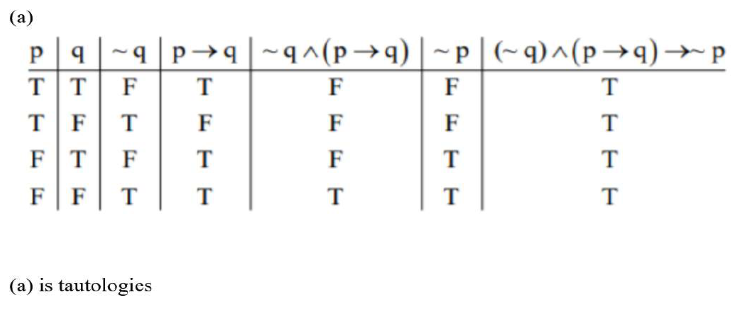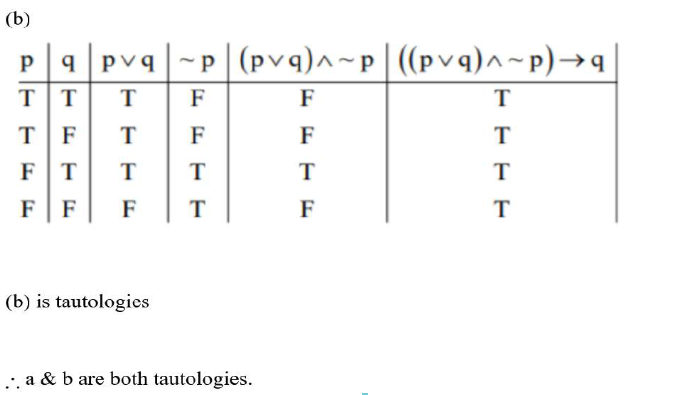# For the statements p and q, consider the following compound statements:Question:

For the statements $\mathrm{p}$ and $\mathrm{q}$, consider the following compound statements:

(a) $(\sim q \wedge(p \rightarrow q)) \rightarrow \sim p$

(b) $((\mathrm{p} \vee \mathrm{q})) \wedge \sim \mathrm{P}) \rightarrow \mathrm{P}$

Then which of the following statements is correct?

1. (1) (a) is a tautology but not (b)

2. (2) (a) and (b) both are not tautologies.

3. (3) (a) and (b) both are tautologies.

4. (4) (b) is a tautology but not (a).

Correct Option: , 3

Solution: